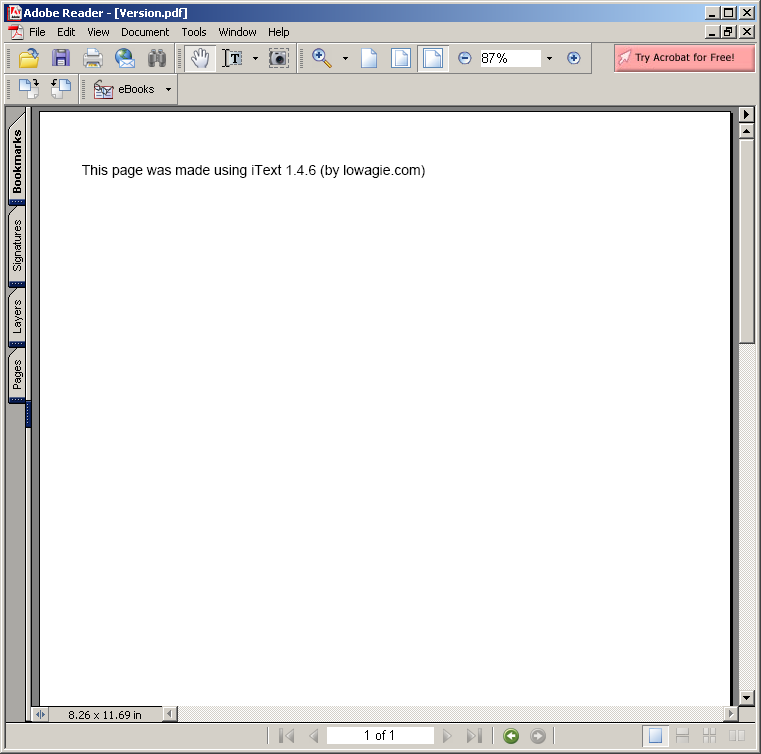# Reverse Polish Notation : Algorithms « Collections Data Structure « Java

Reverse Polish Notation

```
//package rpn;

import java.util.Stack;
import java.util.Scanner;

/**
* ReversePolishNotation is a simple application which will test several RPN
* equations to make sure the calcRPN method works properly.
*
* @author Alex Laird
* @version 1.0 File: ReversePolishNotation.java Created: Oct 2008
*/
public class ReversePolishNotation {
/**
* This method tests whether the calculated answer is within the acceptable
* bounds of the actual answer to be correct.
*
*            is the correct answer to the RPN equation
*            is the calculated answer to the RPN from calcRPN
*/
public static void checkPrecision(Double actAnswer, Double calcAnswer) {
if (Math.abs(calcAnswer - actAnswer) < 0.000001) {
return;
} else {
System.out
.println("The calculated answer was not within 0.000001 of the actual answer.");
}
}

/**
* This method tests to see whether the value of a String is a legal RPN
* mathematical operator or not.
*
* @param next
*            is the value to be tested
* @return whether the next value is a mathematical operator or not
*/
public static boolean nextIsOperator(String next) {
return (next.equals("+") || next.equals("-") || next.equals("*") || next
.equals("/"));
}

/**
* This method will calculate the answer of the given Reverse Polar Notation
* equation. All exceptions are thrown to the parent for handling.
*
* @param input
*            is the equation entered by the user
* @throws EmptyRPNException
*             when there is no RPN equation to be evaluated.
* @throws RPNDivideByZeroException
*             when the RPN equation attempts to divide by zero.
* @throws RPNUnderflowException
*             when the RPN equation has a mathematical operator before
*             there are enough numerical values for it to evaluate.
* @throws InvalidRPNException
*             when the RPN equation is a String which is unable to be
*             manipulated.
* @throws RPNOverflowException
*             when the RPN equation has too many numerical values and not
*             enough mathematical operators with which to evaluate them.
* @return the top item of the stack; the calculated answer of the Reverse
*         Polish Notation equation
*/
public static double calcRPN(String input) {
// eliminate any leading or trailing whitespace from input
input = input.trim();
// scanner to manipulate input and stack to store double values
String next;
Stack<Double> stack = new Stack<Double>();
Scanner scan = new Scanner(input);

// loop while there are tokens left in scan
while (scan.hasNext()) {
// retrieve the next token from the input
next = scan.next();

// see if token is mathematical operator
if (nextIsOperator(next)) {
// ensure there are enough numbers on stack
if (stack.size() > 1) {
if (next.equals("+")) {
stack.push((Double) stack.pop() + (Double) stack.pop());
} else if (next.equals("-")) {
stack.push(-(Double) stack.pop() + (Double) stack.pop());
} else if (next.equals("*")) {
stack.push((Double) stack.pop() * (Double) stack.pop());
} else if (next.equals("/")) {
double first = stack.pop();
double second = stack.pop();

if (first == 0) {
System.out
.println("The RPN equation attempted to divide by zero.");
} else {
stack.push(second / first);
}
}
} else {
System.out
.println("A mathematical operator occured before there were enough numerical values for it to evaluate.");
}
} else {
try {
stack.push(Double.parseDouble(next));
} catch (NumberFormatException c) {
System.out
.println("The string is not a valid RPN equation.");
}
}
}

if (stack.size() > 1) {
System.out
.println("There too many numbers and not enough mathematical operators with which to evaluate them.");
}

return (Double) stack.pop();
}

/**
* The main method from which the program executes; it handles all testing
* and exception handling.
*
* @param args
*            unused
*/
public static void main(String[] args) {
String[] equations = new String;
Double[] answers = new Double;
double answer = 0.0;

// this equation will pass
equations = "23.3 5 16.2 + 8 * -";
// this equation will fail with an EmptyRPNException
equations = null;
// this equation will fail with an EmptyRPNException
equations = "   ";
// this equation will fail with an InvalidRPNException
equations = "Hello world!";
// this equation will fail with a RPNUnderflowException
equations = "12 * 3 15 18.723 - + 52 /";
// this equation will fail with a RPNDivideByZeroException
equations = "52.2 12 + 17 - 9.7 10 0 / + -";
// this equation will fail with a RPNOverflowException
equations = "12.2 17 / 33.333 - 12";
// this equation will pass
equations = "2 3 / 3 2 / *";

// loop until all test values evaluated
for (int i = 0; i < equations.length; i++) {
System.out.println("Equation #" + (i + 1) + ": " + equations[i]
+ ".");
// call method to calculate answer

// ensure that the calculated answer is within 0.000001 of the

System.out.println("Passed! The calculated value of "
+ equations[i] + " is " + answer + ".\n");
}
}
}

```

### Related examples in the same category

 1 Anagrams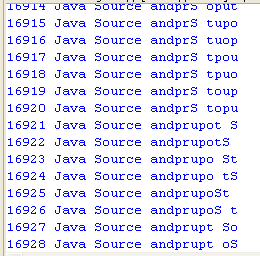2 Hanoi puzzle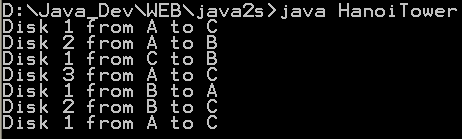3 Fibonacci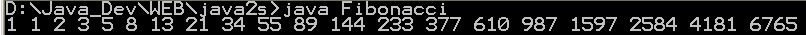4 Sieve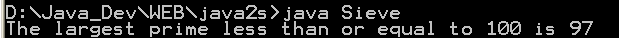5 Find connections using a depth-first search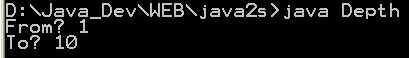6 Find connections using hill climbing. 7 Find optimal solution using least-cost 8 Find the lost keys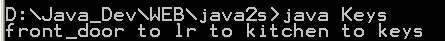9 Compute the area of a triangle using Heron's Formula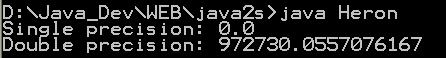10 Compute prime numbers 11 Print a table of fahrenheit and celsius temperatures 1 12 Print a table of fahrenheit and celsius temperatures 2 13 Print a table of Fahrenheit and Celsius temperatures 3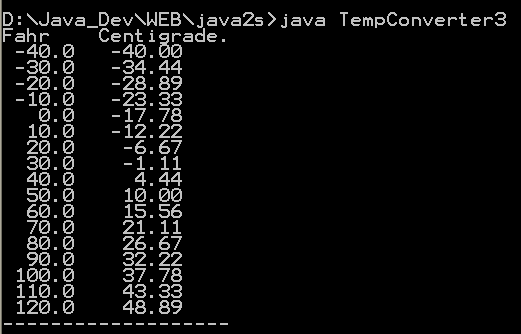14 Soundex - the Soundex Algorithm, as described by Knuth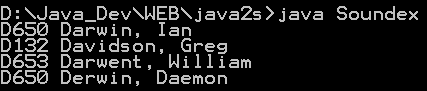15 A programmable Finite State Machine implementation. 16 An extendable Graph datastructure. 17 Utilities for flop (floating-point operation) counting. 18 LU Decomposition 19 Permutator test 20 implements the LZF lossless data compression algorithm 21 Linear Interpolation 22 Utility class for generating the k-subsets of the numbers 0 to n 23 Version